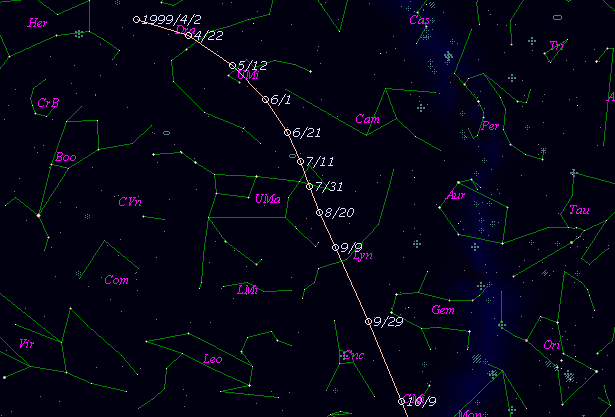# C/1999 J3 ( LINEAR )###Profile

 Discovery Date May 12, 1999 Magnitude 18.8 mag Discoverer Lincoln Laboratory Near-Earth Asteroid Research project

###Pictures###Orbital Elements

```   The following improved orbital elements by Kenji Muraoka, are from
164 observations 1999 May 12  to  Dec. 6, including 9 Planets, Moon
and 5 minor planets perturbations and non-gravitational effect of
style II.    The mean residual is +/- 0.73 arc seconds.

Epoch  =  1999 Sept. 19.0  TT      JDT = 2451440.5
T  =  1999 Sept. 20.16617      +/- 0.00020 (m.e.) TT
Peri. =  161.97954                +/- 0.00019
Node  =  228.97904                +/- 0.00009   (2000.0)
Incl. =  101.65622                +/- 0.00008
q  =    0.9768108              +/- 0.0000035 AU
e  =    0.9993830              +/- 0.0000151
1/a  =   +0.0006317              +/- 0.0000154 1/AU
A1  =   +2.21                   +/- 0.13
A2  =   -0.6575                 +/- 0.1095
( P  =  62986 years )
```

###Finding Charts###Magnitudes Graph

```        m1 = 12.0 + 5 log d + 10.0 log r  [    ,-100]  (             - 1999 June 12)
m1 =  8.8 + 5 log d + 15.0 log r  [-100,  30]  (1999 June 12 - 1999 Oct. 20)
m1 =  9.0 + 5 log d +  5.0 log r  [  30,  75]  (1999 Oct. 20 - 1999 Dec.  4)
m1 =  5.6 + 5 log d + 22.0 log r  [  75,    ]  (1999 Dec.  4 -             )
```##### The orbital elements are calculated by Kenji Muraoka. The charts are made with StellaNavigator Ver.2.0 for Windows (AstroArts / ASCII). The magnitudes graphs are made with Comet for Windows.